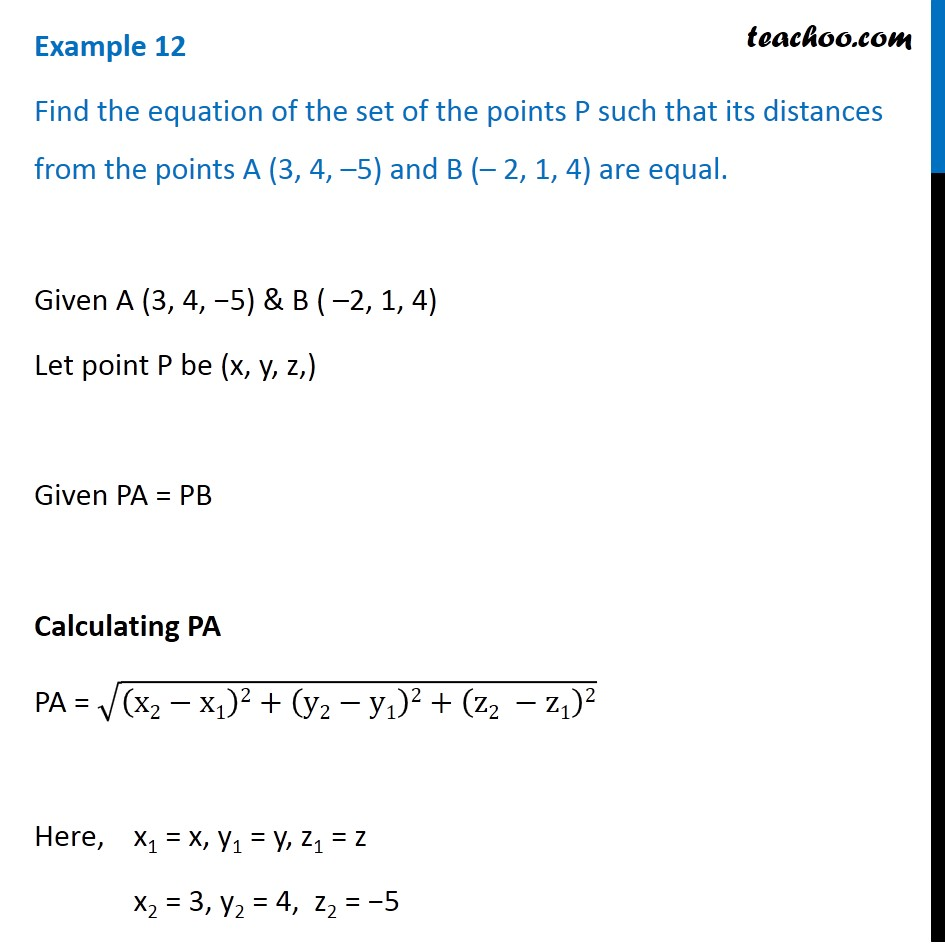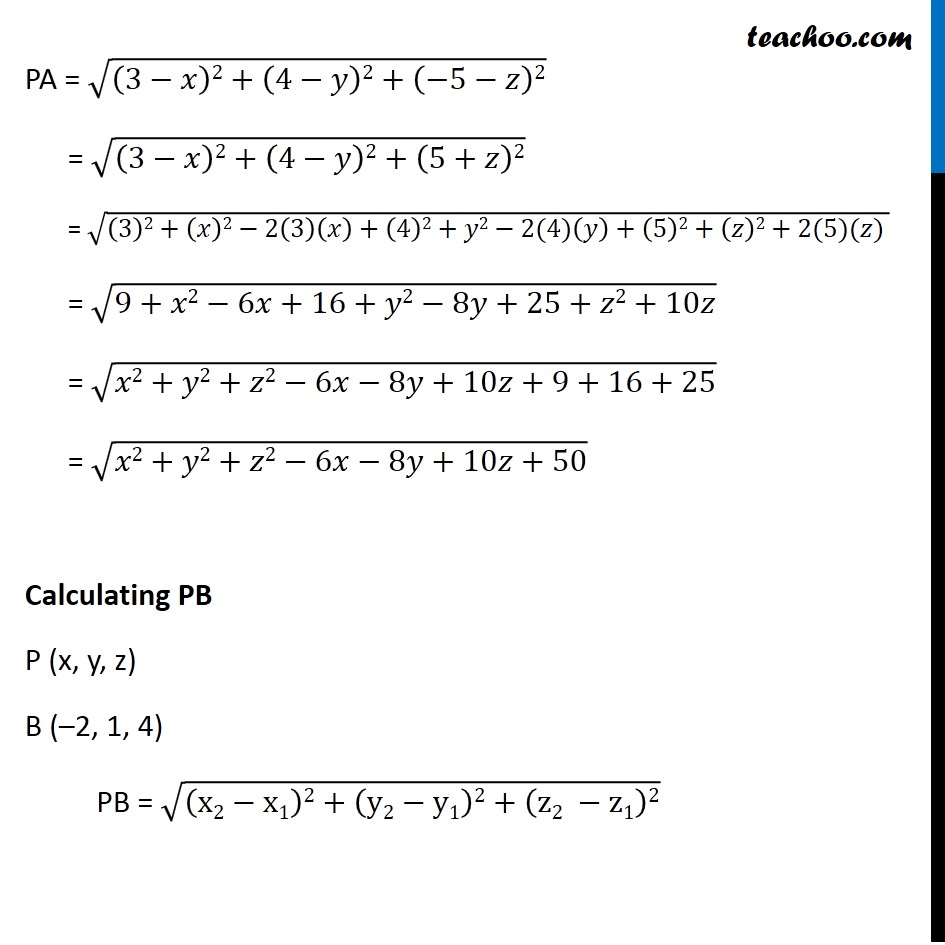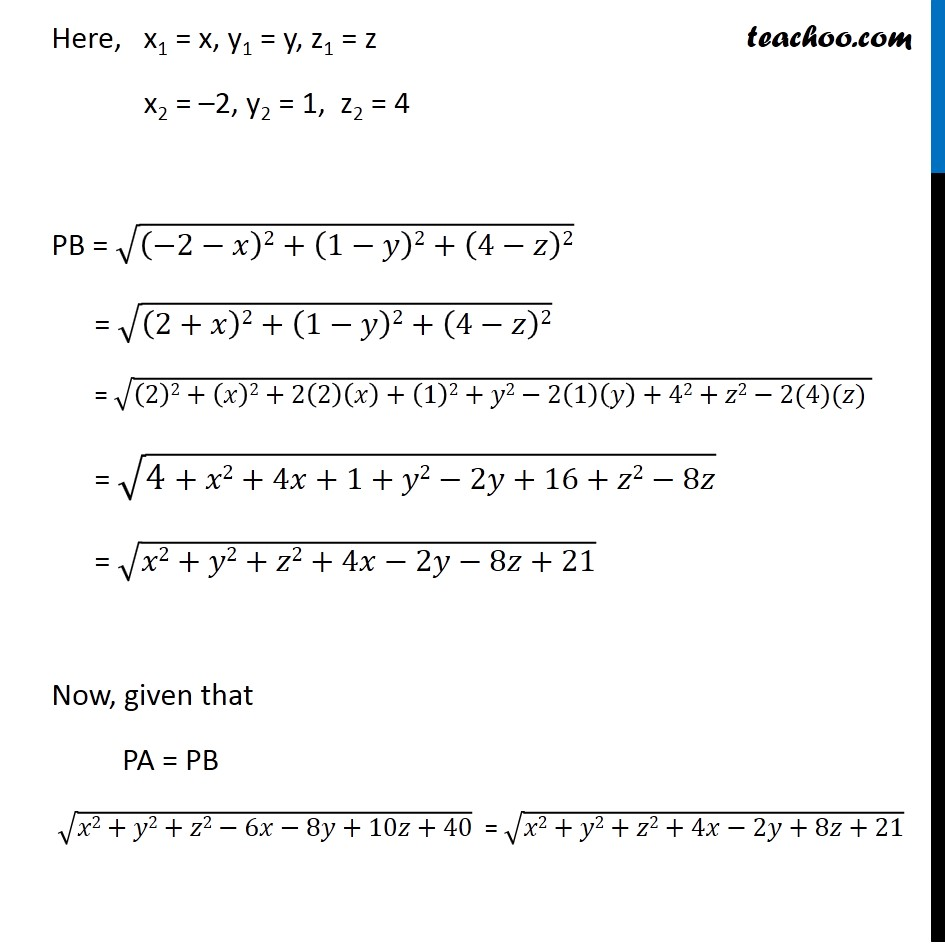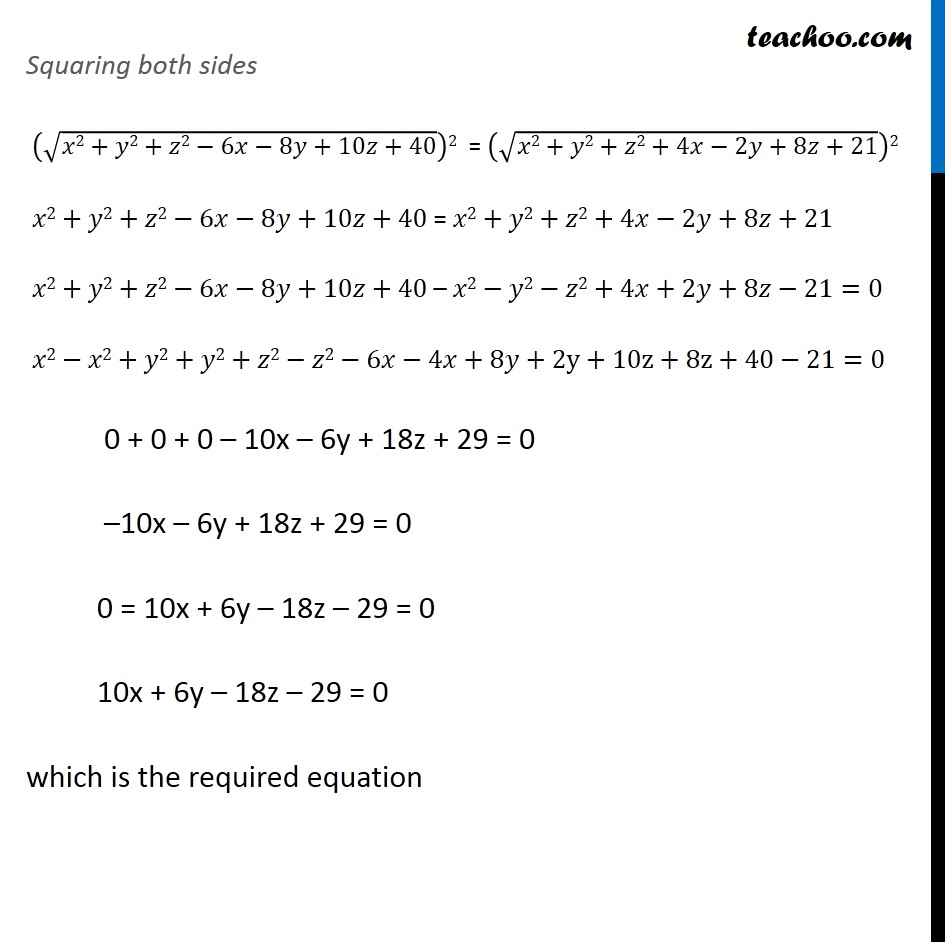Examples

Chapter 11 Class 11 - Intro to Three Dimensional Geometry
Serial order wiseLearn in your speed, with individual attention - Teachoo Maths 1-on-1 Class

### Transcript

Example 8 Find the equation of the set of the points P such that its distances from the points A (3, 4, –5) and B (– 2, 1, 4) are equal. Given A (3, 4, −5) & B ( –2, 1, 4) Let point P be (x, y, z,) Given PA = PB Calculating PA PA = √((x2−x1)2+(y2−y1)2+(z2 −z1)2) Here, x1 = x, y1 = y, z1 = z x2 = 3, y2 = 4, z2 = −5 PA = √((3−𝑥)2+(4−𝑦)2+(−5−𝑧)2) = √((3−𝑥)2+(4−𝑦)2+(5+𝑧)2) = √((3)2+(𝑥)2−2(3)(𝑥)+(4)2+𝑦2−2(4)(𝑦)+(5)2+(𝑧)2+2(5)(𝑧) ) = √(9+𝑥2−6𝑥+16+𝑦2−8𝑦+25+𝑧2+10𝑧) = √(𝑥2+𝑦2+𝑧2−6𝑥−8𝑦+10𝑧+9+16+25) = √(𝑥2+𝑦2+𝑧2−6𝑥−8𝑦+10𝑧+50) Calculating PB P (x, y, z) B (–2, 1, 4) PB = √((x2−x1)2+(y2−y1)2+(z2 −z1)2) Here, x1 = x, y1 = y, z1 = z x2 = –2, y2 = 1, z2 = 4 PB = √((−2−𝑥)2+(1−𝑦)2+(4−𝑧)2) = √((2+𝑥)2+(1−𝑦)2+(4−𝑧)2) = √((2)2+(𝑥)2+2(2)(𝑥)+(1)2+𝑦2−2(1)(𝑦)+42+𝑧2−2(4)(𝑧) ) = √(4+𝑥2+4𝑥+1+𝑦2−2𝑦+16+𝑧2−8𝑧) = √(𝑥2+𝑦2+𝑧2+4𝑥−2𝑦−8𝑧+21) Now, given that PA = PB √(𝑥2+𝑦2+𝑧2−6𝑥−8𝑦+10𝑧+40) = √(𝑥2+𝑦2+𝑧2+4𝑥−2𝑦+8𝑧+21) Squaring both sides (√(𝑥2+𝑦2+𝑧2−6𝑥−8𝑦+10𝑧+40))2 = (√(𝑥2+𝑦2+𝑧2+4𝑥−2𝑦+8𝑧+21))2 𝑥2+𝑦2+𝑧2−6𝑥−8𝑦+10𝑧+40 = 𝑥2+𝑦2+𝑧2+4𝑥−2𝑦+8𝑧+21 𝑥2+𝑦2+𝑧2−6𝑥−8𝑦+10𝑧+40 – 𝑥2−𝑦2−𝑧2+4𝑥+2𝑦+8𝑧−21=0 𝑥2−𝑥2+𝑦2+𝑦2+𝑧2−𝑧2−6𝑥−4𝑥+8𝑦+2y+10z+8z+40−21=0 0 + 0 + 0 – 10x – 6y + 18z + 29 = 0 –10x – 6y + 18z + 29 = 0 0 = 10x + 6y – 18z – 29 = 0 10x + 6y – 18z – 29 = 0 which is the required equation